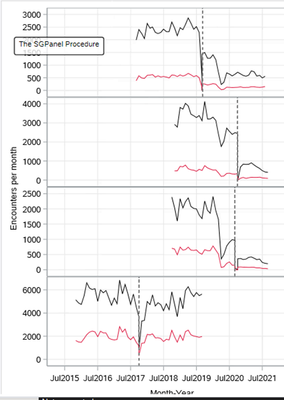## Adding a label to refline in proc sgpanel when refline is a date var

Hello, I am using proc sgpanel to plot encounters over time for 2 variables (disease rates) across 4 groups (hospitals).  Each hospital has a different date for when they switch health records, which I've stored in the variable 'mgen_date'.  The reference line shows up fine, but I can't figure how to apply a label to it.  When I try the code below it doesn't apply the label and gives the warning: "

```proc sgpanel data=XXXX;
where fisc_monyr >= '01Nov2015'd;
panelby hotel / layout=rowlattice onepanel
novarname uniscale=column;
series x=fisc_monyr y=xxx / legendlabel='Any XXX' lineattrs=(color=black);
series x=fisc_monyr y=yyy / legendlabel='YYY XXX' lineattrs=(color=crimson);
colaxis grid
values=('01Nov2015'd to '01Oct2021'd)
label="Month-Year"
display=all interval=semiyear  valuesrotate=diagonal notimesplit;
rowaxis grid
label="Cars per month";
format fisc_monyr monyy..;
refline tanker_date / axis=x label="Mardi Gras Start" lineattrs=(color=gray
pattern=shortDash
thickness=1)
transparency=0.1;
run;```

NOTE: CURVELABEL='MGEN Start' is invalid. The option value cannot be a string when a column of reference lines is plotted. The label will not be drawn."

Here is the graph:I also have questions about how to format the x-axis, but I'll save that for later.  Thanks so much!

Ben

1 ACCEPTED SOLUTION

Accepted Solutions

## Re: Adding a label to refline in proc sgpanel when refline is a date var

Per the documentation of the REFLINE statement for SGpanel:

a text string for the label value.

 Restriction The label string does not apply when a variable is used for the REFLINE value. In that situation, the label string is ignored and a message is written to the log.

If you want to add other text then you could add a variable to the data set to use as the Label= variable in the refline. That value could be different for each Panelby value. One way:

```data plotclass;
set sashelp.class;
if sex='F' then do;
refage=12;
reflabel='Female Refline';
end;
else do;
refage=13;
reflabel='Male reference';
end;
run;

proc sgpanel data=plotclass;
panelby sex;
scatter x=age y=weight;
refline refage /axis=x label=reflabel;
run;```
2 REPLIES 2

## Re: Adding a label to refline in proc sgpanel when refline is a date var

Per the documentation of the REFLINE statement for SGpanel:

a text string for the label value.

 Restriction The label string does not apply when a variable is used for the REFLINE value. In that situation, the label string is ignored and a message is written to the log.

If you want to add other text then you could add a variable to the data set to use as the Label= variable in the refline. That value could be different for each Panelby value. One way:

```data plotclass;
set sashelp.class;
if sex='F' then do;
refage=12;
reflabel='Female Refline';
end;
else do;
refage=13;
reflabel='Male reference';
end;
run;

proc sgpanel data=plotclass;
panelby sex;
scatter x=age y=weight;
refline refage /axis=x label=reflabel;
run;```

## Re: Adding a label to refline in proc sgpanel when refline is a date var

This worked! I wouldn't have thought of this. Thanks so much for your time and expertise, ballardw
Discussion stats
• 2 replies
• 478 views
• 0 likes
• 2 in conversation